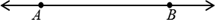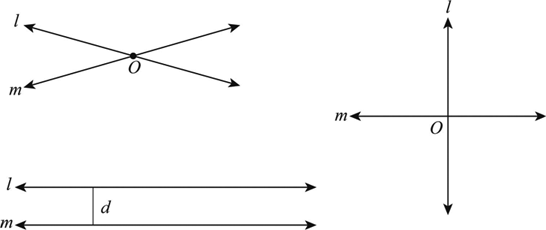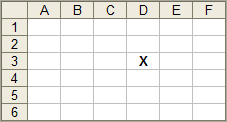# CBSE

## NCERT Solutions for Class 9 Maths Chapter 10 – Circles

2018-10-24T11:27:02+00:00 Categories: NCERT Solution For Class 9 Maths||

Circle, it is one of the basic shapes which we come across in our day to day life. The distance between the center of the circle and the edges remains the same from any given point on the edge. It is round in shape having a definite circumference. IN other words you can all a […]

## NCERT Solutions for Class 9 Maths Chapter 9 – Areas of Parallelograms and Triangles

2018-10-24T09:30:07+00:00 Categories: NCERT Solution For Class 9 Maths||

Class 9 Maths chapter 9 solutions pdf has a number of questions on area of triangle and area of parallelogram. Practice the solutions as many times as possible to master the chapter!

## Area of Triangle:

A triangle has three sides which are joined to each other. A triangle in which of the angle is 90 degrees […]

## NCERT Solutions for Class 9 Maths Chapter 8 – Quadrilaterals

2018-10-24T09:23:53+00:00 Categories: NCERT Solution For Class 9 Maths||

A Quadrilateral has four-sides, it is 2-dimensional (a flat shape), closed (the lines join up), and has straight sides.

• A quadrilateral has 4 sides, 4 angles and 4 vertices.
• A quadrilateral can be regular or irregular.
• The sum of all the interior angles of a quadrilateral is 360 degree.

## Properties of Parallelogram

There are six important properties of parallelograms to acknowledge:

[…]

## NCERT Solutions for Class 9 Maths Chapter 7 – Triangles

2018-10-24T11:39:43+00:00 Categories: NCERT Solution For Class 9 Maths||

A triangle has three sides and three angles. The three angles always add to 180 degree. There are three special names given to triangles that tell how many sides (or angles) are equal.

There can be 32 or no equal sides/angles:

## Equilateral

• Three equal sides
• Threeequal angles, always 60°

## Isosceles

• Two equal sides
• Two equal angles

## Scalene

• No equal sides
• No equal angles

Triangles […]

## NCERT Solutions for Class 9 Maths Chapter 6 – Lines and Angles

2018-10-24T09:15:38+00:00 Categories: NCERT Solution For Class 9 Maths||

## Line

A line has neither initial nor terminal points with indefinite length.The pairs of lines are nothing but the two lines, which may be intersects or parallel or perpendicular as shown below:The […]

## NCERT Solutions for Class 9 Maths Chapter 5 – Introduction to Euclid’s Geometry

2018-10-24T09:15:15+00:00 Categories: NCERT Solution For Class 9 Maths||

Euclidean geometry is the study of plane and solid figures on the basis of axioms and theorems employed by the Greek mathematician Euclid. In its rough outline, Euclidean geometry is the plane and solid geometry commonly taught in secondary schools. For details
class 9 maths chapter 5 solutions can be referred to.

## Euclid’s axioms

·         Things which […]

## NCERT Solutions for Class 9 Maths Chapter 4 – Linear Equations in Two Variables

2018-10-24T11:59:33+00:00 Categories: NCERT Solution For Class 9 Maths||

Linear equations with two variables class 9 covers introduction and methods of solving linear equations with two variables

We all know about linear equation with one variable can be represented as

ax = b where a, b are constants and x is a variable.

Linear Equations with Two Variables will have two variables, it can be represented as

ax+by […]

## NCERT Solutions for Class 9 Maths Chapter 3 – Co-ordinate Geometry

2018-10-24T11:56:27+00:00 Categories: NCERT Solution For Class 9 Maths||

## Coordinate Geometry

• Introduction
• Cartesian System
• Plotting a Point in the Plane if its Coordinates are Given and
• Summary

The position of an object on a plane can be defined with the help of coordinate geometry.

## What are coordinates?Consider a plane which is continuous in both the directions. […]

## NCERT Solutions for Class 9 Maths Chapter 2 – Polynomials

2018-10-24T11:55:11+00:00 Categories: NCERT Solution For Class 9 Maths||

If any given expression has more than three terms, then the expression is called as polynomials.

## Types of Polynomials:

The polynomials can be classified based on the number of terms as given below

### Monomial:

The polynomial with only one term is monomial

Eg: It’s a number or a number with variable

### Binomial:

The polynomial with only two terms is Binomial

Eg: It […]

## NCERT Solutions for Class 9 Maths Chapter 1 – Number Systems

2018-10-24T11:46:58+00:00 Categories: NCERT Solution For Class 9 Maths||

NCERT solutions for class 9 maths chapter 1 pdf, Number system covers the major topics rational numbers, irrational numbers and decimal expansion of real numbers.

## Rational Numbers:

The rational numbers can be positive, negative, in the form of fraction or zero.

The word rational is derived from the word ration, which can be given as fraction. Ratio compares […]

Hello